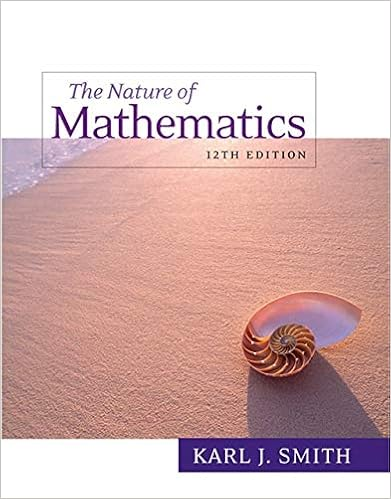# Practice Problems - Math 230 Practice Problems for Test II...

• Notes
• 5

This preview shows page 1 - 3 out of 5 pages.

##### We have textbook solutions for you!
The document you are viewing contains questions related to this textbook.The document you are viewing contains questions related to this textbook.
Chapter E / Exercise 19
Nature of Mathematics
SmithExpert Verified
Math 230, Practice Problems for Test IISpace Curves, Curvature and Arclength1. Match each of the vector valued functions to its graph.4. (9 points) Match each of the vector valued functions to its graph.(A)r(t) =h8 cos(4t),8 sin(4t), ti(B)r(t) =h8 cos(4t), t,8 sin(4t)i(C)r(t) =htcos(4t), t, tsin(4t)i(D)r(t) =h2 +t,0,2ti(E)r(t) =ti+tj+ (2-t2)k(F)r(t) = sint2i+ 5 cost2j+tkMath 214-3 Test # 1Spring Quarter 2003Page 5 of 11(A)r(t) =<8 cos(4t),8 sin(4t), t >(B)r(t) =<8 cos(4t), t,8 sin(4t)>(C)r(t) =< tcos(4t), t, tsin(4t)>(D)r(t) =<2 +t, t,8,2t >(E)r(t) =ti+tj+ (2-t2)k(F)r(t) = sint2i+ 5 cost2j+tk(I)(II)(III)(IV)(V)(V I)1
##### We have textbook solutions for you!
The document you are viewing contains questions related to this textbook.The document you are viewing contains questions related to this textbook.
Chapter E / Exercise 19
Nature of Mathematics
SmithExpert Verified
2. Find the curvatureκof the curve~r(t) =h2 sint,3t,2 costiwhent=π2.3. The parametric equations of a moving point arex(t) = 2 sin 3t,y(t) =-8t,z(t) = 2 cos 3t.(a) Find its velocity, speed, and acceleration at all times.(b) Find the distance traveled by the point fromt= 0 tot= 5.(c) Find the equation of the tangent line to the curve at the point whent= 0.4. A body moves in space so that at timetthe acceleration of the body is given by thevectora(t) = (cost)i+ (sint)j-gk,wheregis aconstantscalar value. Att= 0 thebody is at the origin and has velocity 4j.(a) What is the position of the body whent=π2?
•••